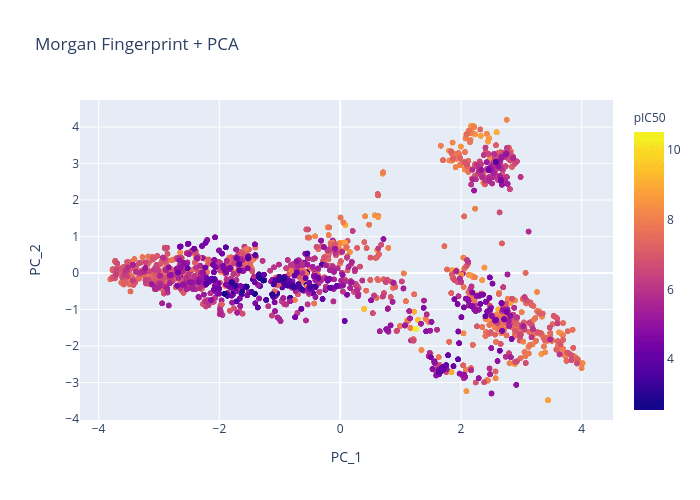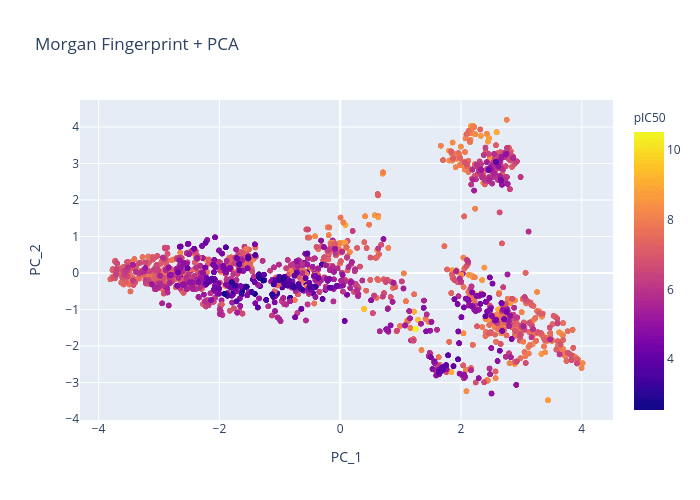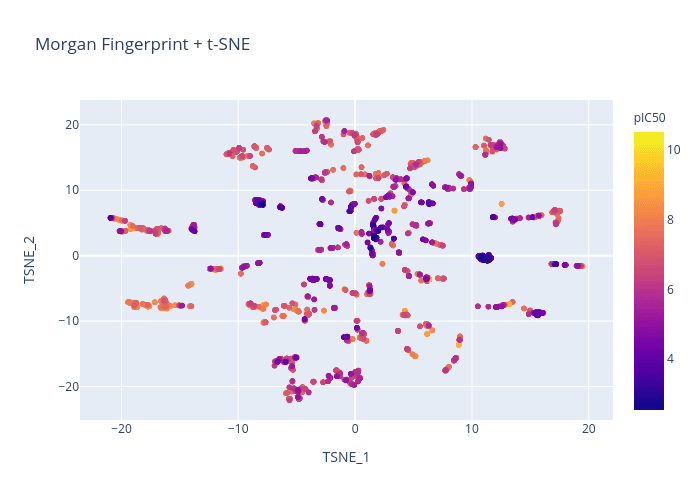# Visualizing the chemical space

Jun 29, 2022After creating models for drug classification, we need a practical way of visualizing our chemical space and verifying our results. This guide will walk you through how to generate an interactive plotly graph of chemicals that renders 2D images of molecules on hover. A good library for this is molplotly, and the below tutorial teaches you how to write and customize your own code for this.

We will cover two different types of chemical representations (Morgan fingerprint and RDKit 2D molecular descriptors), two different techniques for dimension reduction (PCA and t-SNE), generating a scatterplot in plotly, and creating an interactive Dash app which displays structures on hover. We’ll be using the BACE dataset from MoleculeNet. All of the code is located in our “Putting Everything Together” section at the end, but we recommend reading through the sections to fully understand how everything works.Let’s get started! 🙂

# Chemical representations

### Morgan Fingerprints

RDKit provides a function for converting SMILES into Morgan fingerprints that requires the fingerprint radius and number of bits. It is common to use a radius of 2 or 3 and at least 1024 bits. Higher bit values will allow you to retain more information about the molecule. In order to perform PCA or t-SNE, we convert the fingerprint into a 1d numpy array.

``````from rdkit import Chem, DataStructs
from rdkit.Chem import AllChem
import numpy as np

def fp_from_smiles(smiles):
mol = Chem.MolFromSmiles(smiles)
fp = AllChem.GetMorganFingerprintAsBitVect(mol, 2, nBits=2048) # generate MF as bit vector
fp = np.frombuffer(fp.ToBitString().encode(), 'u1') - ord('0') # convert bit vector to 1d numpy array
return fp``````

### RDKit 2D Normalized Descriptor

Descriptastorus is a package for that allows us to efficiently generate a wide range of molecular descriptors from chemical SMILES. Luckily for us, they are also able to calculate and normalize the 2D molecular descriptors provided in RDKit.

The output of processing a SMILES on the RDKit2DNormalized generator is a 1d array.

``````from rdkit import Chem, DataStruct
from descriptastorus.descriptors import rdNormalizedDescriptors

def rdnd_from_smiles(smiles):
generator = rdNormalizedDescriptors.RDKit2DNormalized()
rdnd = generator.process(smiles)
return rdnd``````

# Dimensionality Reduction

### Principal Component Analysis

The scikit-learn package provides a function to perform PCA. We just need to pass in our dataset as an array of the molecules’ chemical representations (chem_rep_list) and specify the number of components. To plot this on a graph, we are limited to 2 or 3 components.

``````from rdkit import Chem, DataStructs
from sklearn.decomposition import PCA

def pca_df(chem_rep_list):
pca = PCA(n_components=2)
pca_arr = pca.fit_transform(chem_rep_list)
return pd.DataFrame(pca_arr, columns=["Component 1", "Component 2"])``````

### t-Distributed Stochastic Neighborhood Embedding

Alternatively, we can use t-SNE to reduce the number of dimensions. However, this technique is more computationally heavy, so it’s recommended to use PCA first to reduce the data down to around 50 dimensions.

The t-SNE function has many parameters. We recommend modifying them within a range as different values can give dramatically different results.

``````from rdkit import Chem, DataStructs
from sklearn.manifold import TSNE

def tsne_df(chem_rep_list):
pca_50 = PCA(n_components=50)
pca_result_50 = pca_50.fit_transform(chem_rep_list)
pca_50_variance = pca_50.explained_variance_ratio_
tsne = TSNE(n_components=2, verbose=0, perplexity=40, n_iter=300)
tsne_results = tsne.fit_transform(pca_result_50)mol = Chem.MolFromSmiles(smiles)
return pd.DataFrame(tsne_results, columns=["TSNE-1", "TSNE-2"])``````

### Graphing with Plotly

We can use any of the chemical representations above with any of the dimension reduction techniques. Let’s combine some of the code we’ve written to create a function that allows us to generate a plotly graph.

``````from rdkit import Chem, DataStructs
from sklearn.manifold import TSNE

def graph_chemical_space(
df: pd.DataFrame,
smiles_col: str = "SMILES",
color_col: str = None,
id_col: str = None,
chem_rep: str = "rdkit",
dim_reduction: str = "TSNE",
graph_title: str = None
):
"""
Written by Melissa Du, Oloren AI
df : pandas.DataFrame object
a pandas dataframe that contains the data plotted in fig.
smiles_col : str, optional
name of the column in df containing the smiles plotted in fig (default 'SMILES').
If provided as a list, will add a slider to choose which column is used for rendering the structures.
color_col : str, optional
name of the column in df that will specify the point colors plotted in fig (default None)
id_col : str, optional
name of the column in df that will specify id of the points plotted in fig (default None)
chem_rep : "rdkit" | "mf" | "oc" , optional
name of the desired chemical representation
dim_reduction : "PCA" | "TSNE" , optional
name of desired dimension reduction technique
graph_title : str, optional
title of graph
"""

assert chem_rep in ['rdkit', 'mf', 'oc'], "Please enter a valid chemical representation"
assert dim_reduction in ["PCA", "TSNE"], "Please enter a valid dimension reduction technique"
assert smiles_col in df, "The specified smiles_col is not in the dataframe"

funcs = {
"rdkit" : rdnd_from_smiles,
"mf" : fp_from_smiles,
"TSNE": tsne_df,
"PCA": pca_df
}

mol_list = []
# convert smiles to desired chemical representation
for smiles in df[smiles_col]:
mol_list.append(funcs[chem_rep](smiles))
# apply desired dimension reduction technique
rd_df = funcs[dim_reduction](mol_list)
# populate dataframe with color and id columns
rd_df = rd_df.join(df[smiles_col])
if id_col is not None:
rd_df = rd_df.join(df[id_col])
if color_col is not None:
rd_df = rd_df.join(df[color_col])

fig = px.scatter(rd_df, x='Component 1', y='Component 2', color=color_col, title=graph_title)
return fig``````

We will use the BACE dataset and attempt to generate a chemical visualization that distinguishes molecules by pIC50.

``````from rdkit import Chem, DataStructs
import pandas as pd

file_name = 'bace.csv'

Here is a quick comparison of the different chemical representations and dimension reduction techniques:

``graph_chemical_space(df, smiles_col='mol', color_col='pIC50', id_col='CID', chem_rep='mf', dim_reduction='PCA', graph_title='Morgan Fingerprint + PCA')``Figure 1. Morgan Fingerprint + PCA

``graph_chemical_space(df, smiles_col='mol', color_col='pIC50', id_col='CID', chem_rep='mf', dim_reduction='TSNE', graph_title='Morgan Fingerprint + TSNE')``Figure 2. Morgan Fingerprint + t-SNE

``graph_chemical_space(df, smiles_col='mol', color_col='pIC50', id_col='CID', chem_rep='rdkit', dim_reduction='PCA', graph_title='RDKit Descriptors + PCA')``Figure 3. RDKit + PCA

``fig = graph_chemical_space(df, smiles_col='mol', color_col='pIC50', id_col='CID', chem_rep='rdkit', dim_reduction='TSNE', graph_title='RDKit Descriptors + TSNE')``Figure 4. RDKit + t-SNE

# Creating Dash App

Now that we’ve generated some graphs, we’re onto the last step of this process: displaying 2D images of the molecules on hover! For this, we will be using Dash, a python framework for creating interactive web applications.

### Basic Dash Layout

A Dash app is composed of a tree of nested components that can take the form of

1. HTML elements (`dash.html`): basic components like headers, paragraphs, images

2. Dash Core Component elements (`dash.dcc`): higher-level, interactive components like graphs, drop-downs, tooltips

All components can be given an identifying id for future reference. HTML components can be styled with a CSS dictionary. Our app will be composed of a DCC Graph component that renders our plotly graph and a DCC Tooltip that allows the user to point to a precise location on the graph.

The following code generates a basic Dash app that displays the plotly graph above without any hover functionality. Note that we are using JupyterDash, which gives us the option to display the graph inline in a Jupyter notebook in addition to externally on a web browser.

Setting `debug=True` in `app.run_server` allows the app to update in real-time as changes are being made to the code. Additionally, a port must be specified (default is 8050) and forwarded to display the app interface inline. Make sure you manually forward the port in a Jupyter notebook as the app will not fully render otherwise.

``````from rdkit import Chem, DataStructs
fig = graph_chemical_space(df, smiles_col='mol', color_col='pIC50', id_col='CID', chem_rep='rdkit', dim_reduction='TSNE', graph_title='RDKit Descriptors + TSNE')
fig.update_traces(hoverinfo="none", hovertemplate=None) # remove all previous hover info, no selector or col/row specificed
app = JupyterDash(__name__)
app.layout = html.Div(
[
dcc.Graph(id="graph", figure=fig, clear_on_unhover=True),
dcc.Tooltip(id="graph-tooltip"),
]
)
app.run_server(debug=True, port='8050')``````

### Basic Dash Callback

In order to display molecules on hover, we need to add interactivity to our app. More specifically, we want to add a callback function that updates our Dash interface whenever we hover over a point on the graph.

Callbacks are handled by the `@app.callback` decorator, which which takes in input and output arguments. The inputs and outputs of `@app.callback` are properties of components, which are referenced by their ids.

The decorator causes the function that it wraps to be called anytime there are modifications to the input components. Therefore, the wrapped function must specify how the output should be updated. This function must be written directly after the `@app.callback` decorator (no empty lines) and take in input properties as arguments.

In our case, the input is the hoverData attribute from the Graph component and our output is the data displayed by our Tooltip component.

``````from rdkit import Chem, DataStructs

@app.callback(
Output("graph-tooltip", "show"),
Output("graph-tooltip", "bbox"),
Output("graph-tooltip", "children"),
Input("graph", "hoverData"),
)``````

Now, we have to define a function that describes how our Tooltip is updated in response to changes in the Graph’s hoverData. This function should:

1. Generate a 2D molecule image from a SMILES that can be displayed in an HTML Image component.

2. Display an HTML Div that contains this image, along with the molecule name and any other captions that are specified.

``````def hover(hoverData):
if hoverData is None:
return False, no_update, no_update

pt = hoverData["points"]
bbox = pt["bbox"]
num = pt["pointNumber"]
df_row = df.iloc[num]

# Generate 2D image of molecule
hoverbox = []

if title_col is not None:
hoverbox.append(html.H4(df_row[title_col], style={"font-family": 'Georgia', "fontSize": 14}))
img_str = image_str_from_smiles(df_row[smiles_col], 400)
hoverbox.append(html.Img(src=img_str, style={"width": "100%"}))
if hover_cols is not None:
for col in hover_cols:
hoverbox.append(html.P(f"{col} : {df_row[col]}", style={"font-family": 'Georgia', "fontSize": 12}))

children = [html.Div(hoverbox, style={"width": f"200px", "white-space": "normal"})]

return True, bbox, children``````

## Putting Everything Together

Here is the final code that implements everything we went over. Note that we also added in drop-downs so that the chemical representation and dimension reduction technique can be modified within the Dash app.

``````import base64
from io import BytesIO

import pandas as pd
import numpy as np
from rdkit.Chem.Draw import rdMolDraw2D
from rdkit.Chem import AllChem
from rdkit import Chem
from descriptastorus.descriptors import rdNormalizedDescriptors
from sklearn.decomposition import PCA
from sklearn.manifold import TSNE
from plotly.graph_objects import Figure
import plotly.express as px
from dash import Dash, Input, Output, dcc, html, no_update
from jupyter_dash import JupyterDash

def image_str_from_smiles(smiles, svg_size) -> str:
"""
Takes a SMILES and image size and generates a string
that can be used as the image src in html.
"""
buffered = BytesIO()
d2d = rdMolDraw2D.MolDraw2DSVG(svg_size, svg_size)
d2d.drawOptions().clearBackground = False
d2d.DrawMolecule(Chem.MolFromSmiles(smiles))
d2d.FinishDrawing()
img_str = d2d.GetDrawingText()
buffered.write(str.encode(img_str))
img_str = base64.b64encode(buffered.getvalue())
img_str = f"data:image/svg+xml;base64,{repr(img_str)[2:-1]}"
return img_str

def rdnd_from_smiles(smiles):
"""
Takes in a SMILES and returns a list of the RDKit 2D normalized descriptors
of the molecule.
"""
generator = rdNormalizedDescriptors.RDKit2DNormalized()
rdnd = generator.process(smiles)
return rdnd

def fp_from_smiles(smiles):
"""
Takes in a SMILES and returns a the Morgan Fingerprint representation
of the molecule.
"""
mol = Chem.MolFromSmiles(smiles)
fp = AllChem.GetMorganFingerprintAsBitVect(mol, 2, nBits=2048) # generate MF as bit vector
fp = np.frombuffer(fp.ToBitString().encode(), 'u1') - ord('0') # convert bit vector to 1d numpy array
return fp

def tsne_df(chem_rep_list):
"""
Takes in a list containing the chemical representation of a collection of
molecules and returns a dataframe containing the t-SNE reduction to 2 components.
"""
pca_50 = PCA(n_components=50)
pca_result_50 = pca_50.fit_transform(chem_rep_list)
tsne = TSNE(n_components=2, verbose=0, perplexity=40, n_iter=300)
tsne_results = tsne.fit_transform(pca_result_50)
return pd.DataFrame(tsne_results, columns=["Component 1", "Component 2"])

def pca_df(chem_rep_list):
"""
Takes in a list containing the chemical representation of a collection of
molecules and returns a dataframe containing the PCA reduction to 2 components.
"""
pca = PCA(n_components=2)
pca_arr = pca.fit_transform(chem_rep_list)
return pd.DataFrame(pca_arr,columns=["Component 1","Component 2"])

def add_molecules(df, port, smiles_col = "SMILES", color_col = None, title_col = None, hover_cols = None) -> Dash:
"""
Written by Melissa Du,  Oloren AI

Takes in a dataframe with molecular SMILES and returns a dash app that displays
molecules on hover for a graph of any chemical representation and dimension reduction technique.
---
df : pandas.DataFrame object - contains the data plotted in fig
port : int - the port that the app server uses
smiles_col : str, optional (default 'SMILES') - name of the column in df containing SMILES
title_col : str, optional (default None) - name of the column in df that specifies title in hover box
hover_cols : list[str], optional (default None) - list of column names in df to be included in the hover box
"""
app = JupyterDash(__name__)

app.layout = html.Div(
[
dcc.Tooltip(id="graph-tooltip"),
dcc.Dropdown(id='chem-rep-dd', options=[{'value': 'mf', 'label': 'Morgan Fingerprint'}, {'value': 'rdkit', 'label': 'RDKit 2D Descriptors'}], value='mf'),
dcc.Dropdown(id='dim-red-dd', options=[{'value': 'pca', 'label': 'PCA'}, {'value': 'tsne', 'label': 't-SNE'}], value='pca'),
]
)
@app.callback(
Output("graph", "figure"),
Input("chem-rep-dd", "value"),
Input("dim-red-dd", "value"),
)
def update_graph(chem_rep, dim_red):
print(chem_rep, dim_red)
fig = graph_chemical_space(df, smiles_col=smiles_col, color_col=color_col, id_col=title_col, chem_rep=chem_rep, dim_reduction=dim_red, graph_title=f'{chem_rep} + {dim_red}')
return fig

@app.callback(
Output("graph-tooltip", "show"),
Output("graph-tooltip", "bbox"),
Output("graph-tooltip", "children"),
Input("graph", "hoverData"),
)
def hover(hoverData):
if hoverData is None:
return False, no_update, no_update

pt = hoverData["points"]
bbox = pt["bbox"]
num = pt["pointNumber"]
df_row = df.iloc[num]

hoverbox = []
if title_col is not None:
hoverbox.append(html.H4(df_row[title_col], style={"font-family": 'Georgia', "fontSize": 14}))
img_str = image_str_from_smiles(df_row[smiles_col], 400)
hoverbox.append(html.Img(src=img_str, style={"width": "100%"}))
if hover_cols is not None:
for col in hover_cols:
hoverbox.append(html.P(f"{col} : {df_row[col]}", style={"font-family": 'Georgia', "fontSize": 12}))

children = [html.Div(hoverbox, style={"width": f"200px", "white-space": "normal"})]
return True, bbox, children

print("If you are working on a remote machine, make sure you forward the port number provided in the add molecules function.")
app.run_server(mode='inline', port=port)
return app

def graph_chemical_space(df, smiles_col = "SMILES", color_col = None, id_col = None, chem_rep = "rdkit", dim_reduction = "tsne", graph_title = None) -> Figure:
"""
Written by Melissa Du, Oloren AI

Takes in a dataframe of chemical smiles and properties and generates a plotly figure containing a 2D visualization
using a specified chemical representation and dimention reduction technique.
---
df : pandas.DataFrame object - a pandas dataframe that contains the data plotted in fig
smiles_col : str, optional (default 'SMILES') - name of the column in df containing SMILES
color_col : str, optional (default None) - name of the column in df that specifies the plotted point colors
id_col : str, optional (default None) - name of the column in df that species id of the plotted points
chem_rep : "rdkit" | "mf" | "oc" , optional (default 'rdkit') - name of the desired chemical representation
dim_reduction : "pca" | "tsne" , optional (default 'tsne') - name of desired dimension reduction technique
graph_title : str, optional (default None) - title of graph
port : int - port number, must be forwarded if using a remote machine
"""
assert chem_rep in ['rdkit', 'mf', 'oc'], "Please enter a valid chemical representation"
assert dim_reduction in ["pca", "tsne"], "Please enter a valid dimension reduction technique"
assert smiles_col in df, "The specified smiles_col is not in the dataframe"

funcs = {
"rdkit" : rdnd_from_smiles,
"mf" : fp_from_smiles,
"tsne": tsne_df,
"pca": pca_df,
}

mol_list = []
# convert smiles to desired chemical representation
for smiles in df[smiles_col]:
mol_list.append(funcs[chem_rep](smiles))
# apply desired dimension reduction technique
rd_df = funcs[dim_reduction](mol_list)
# populate dataframe with color and id columns
rd_df = rd_df.join(df[smiles_col])
if id_col is not None:
rd_df = rd_df.join(df[id_col])
if color_col is not None:
rd_df = rd_df.join(df[color_col])

fig = px.scatter(rd_df, x='Component 1', y='Component 2', color=color_col, title=graph_title)
fig.update_traces(hoverinfo="none", hovertemplate=None) # remove all previous hover info, no selector or col/row specificed
fig.update_layout(transition_duration=500)
return fig

file_name = 'bace.csv'